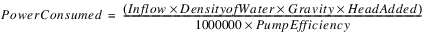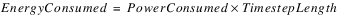Inline Pump Energy
The Inline Pump Energy category is used specify the methods that determine the power consumed by the Inline Pump. The category is dependent on having the Head Lookup method selected. There are two methods: None and Energy Equation.None
This is the default method. It performs no calculations.Energy Equation
The Energy Equation method uses the standard formula to determine the power consumed by the pump.
Slots Specific to This Method
Power Consumed
Type: SeriesSlot
Units: Power
Description: Power consumed by Inline Pump
I/O: Output only
Energy Consumed
Type: SeriesSlot
Units: Energy
Description: Energy consumed by Inline Pump
I/O: Output only
Density of Water
Type: Scalar Slot
Units: Density
Description: Density of the Water
I/O: Input only
Pump Efficiency
Type: ScalarSlot
Units: Decimal
Description: Efficiency of the Inline Pump
I/O: Input only
Method Details
The energy and power consumed by the pump are calculated according to the following formulas.Revised: 01/11/2023